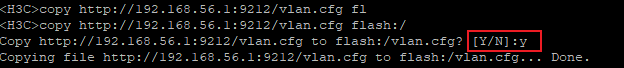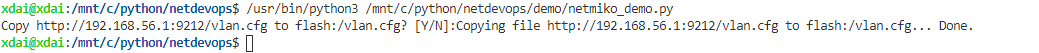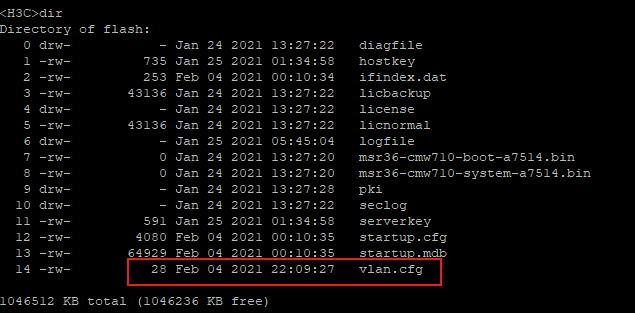## 【python 网络自动化】netmiko——使用 netmiko 进行交互式操作

2021/2/6 22:23:11 文章标签: 测试文章如有侵权请发送至邮箱809451989@qq.com投诉后文章立即删除

# 目录

【python 网络自动化】netmiko——python 使用 netmiko 连接 h3c 交换机网络设备
【python 网络自动化】netmiko——netmiko 常用方法
【python 网络自动化】netmiko——使用 netmiko 进行交互式操作

### 文章目录

• 目录
• copy 文件
• 升级系统

``````# send_command 方法支持的所有参数
def send_command
(self, command_string, expect_string=None, delay_factor=1, max_loops=500, auto_find_prompt=True, strip_prompt=True, strip_command=True, normalize=True, use_textfsm=False, textfsm_template=None, use_ttp=False, ttp_template=None, use_genie=False, cmd_verify=True)
``````

## copy 文件``````from netmiko import ConnectHandler as ch
host = {
'device_type': 'hp_comware',
'host': '192.168.56.20',
'port': 22,
}
cmd = 'copy http://192.168.56.1:9212/vlan.cfg flash:/'
conn = ch(**host)
# 这里要注意使用原始字符串，并对正则表达式中的元字符进行转义
output = send_command(cmd. expect_string=r'\[Y/N\]:')
````````````...
output = conn.send_command(cmd, expect_string=r'\[Y/N\]:')
output += conn.send_command('Y', expect_string=r'>')
print(output)
``````## 升级系统

``````...
output = conn.send_command(cmd, expect_string=r'\[Y/N\]:')
output += conn.send_command('Y', expect_string=r'>', delay_factor=3)
``````

`delay_factor` 的取值 可根据具体的网络情况等因素进行设置，最好预留时间多一点，因为最终 netmiko 检测到预期的提示符后也会退出。

暂无相关的数据...